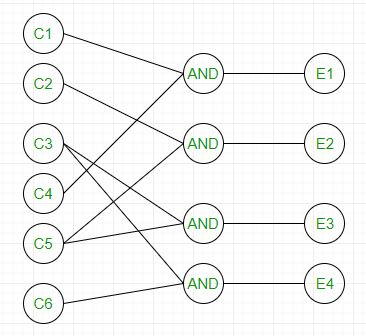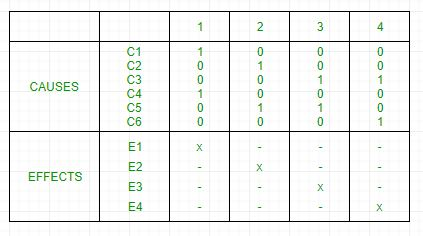Related Articles
Software Engineering | Black box testing
• Difficulty Level : Medium
• Last Updated : 30 Apr, 2019

Prerequisite – Software Testing | Basics
Black box testing is a type of software testing in which the functionality of the software is not known. The testing is done without the internal knowledge of the products.

Black box testing can be done in following ways:

1. Syntax Driven Testing – This type of testing is applied to systems that can be syntactically represented by some language. For example- compilers,language that can be represented by context free grammar. In this, the test cases are generated so that each grammar rule is used at least once.

2. Equivalence partitioning – It is often seen that many type of inputs work similarly so instead of giving all of them separately we can group them together and test only one input of each group. The idea is to partition the input domain of the system into a number of equivalence classes such that each member of class works in a similar way, i.e., if a test case in one class results in some error, other members of class would also result into same error.

The technique involves two steps:

1. Identification of equivalence class – Partition any input domain into minimum two sets: valid values and invalid values. For example, if the valid range is 0 to 100 then select one valid input like 49 and one invalid like 104.
2. Generating test cases –

(i) To each valid and invalid class of input assign unique identification number.
(ii) Write test case covering all valid and invalid test case considering that no two invalid inputs mask each other.

To calculate the square root of a number, the equivalence classes will be:
(a) Valid inputs:

• Whole number which is a perfect square- output will be an integer.
• Whole number which is not a perfect square- output will be decimal number.
• Positive decimals

(b) Invalid inputs:

• Negative numbers(integer or decimal).
• Characters other that numbers like “a”,”!”,”;”,etc.

3. Boundary value analysis – Boundaries are very good places for errors to occur. Hence if test cases are designed for boundary values of input domain then the efficiency of testing improves and probability of finding errors also increase. For example – If valid range is 10 to 100 then test for 10,100 also apart from valid and invalid inputs.

4. Cause effect Graphing – This technique establishes relationship between logical input called causes with corresponding actions called effect. The causes and effects are represented using Boolean graphs. The following steps are followed:

1. Identify inputs (causes) and outputs (effect).
2. Develop cause effect graph.
3. Transform the graph into decision table.
4. Convert decision table rules to test cases.

For example, in the following cause effect graph:It can be converted into decision table like:Each column corresponds to a rule which will become a test case for testing. So there will be 4 test cases.

5. Requirement based testing – It includes validating the requirements given in SRS of software system.

6. Compatibility testing – The test case result not only depend on product but also infrastructure for delivering functionality. When the infrastructure parameters are changed it is still expected to work properly. Some parameters that generally affect compatibility of software are:

1. Processor (Pentium 3,Pentium 4) and number of processors.
2. Architecture and characteristic of machine (32 bit or 64 bit).
3. Back-end components such as database servers.
4. Operating System (Windows, Linux, etc).
My Personal Notes arrow_drop_up